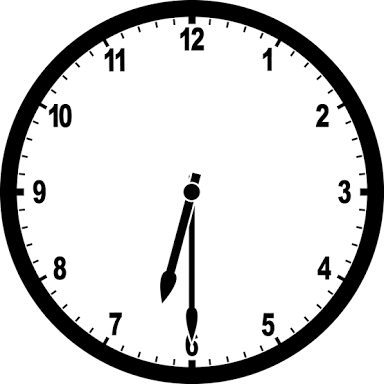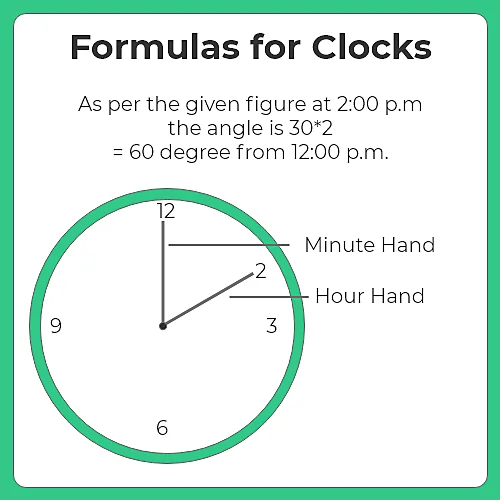# Clock Formulas

## Formula and Basic Concepts of Clock### Clocks Formulas & Properties

• The dial of the clock is circular in shape and is divided into 60 equal minute spaces.
•  60-minute spaces traces an angle of 3600. Therefore, one minute space traverses an angle of 60.
• The hands of a clock are perpendicular when they are 15-minute space apart.
• Hands of a clock are in straight line and are opposite to one another when they are 30 minutes space apart.
• Hands of a clock are perpendicular to each other 22 times in 12 hours and 44 times in 24 hours.
• Hands of the clock are opposite to each other for 11 times in 12 hours and 22 times in a day.
• The minute hand gains 55 minutes over hour hand every hour.A) Angle between hands of a clock

1. When minute hand is behind the hour hand, the angle between the two hands at M minutes past H o’clock.

= 30 [H -(M/5)] + M/2 degree

= 30H – (5.5 x M)

2. In the case where the minute hand is ahead of the hour hand, the angle between the two hands at M minutes past H ‘o clock will be calculated as

= 30 (M/5-H ) -M/2  degree

= 5.5 x M – 30H

B) To calculate x minute space gain by the minute hand over the hour hand,

= x$\frac{60}{55}$

= x$\frac{12}{11}$

C) Both the two hands of the clock will be at the right angles between H and (H+1) o’clock at (5H ± 15)  minutes H<’o clock.

D) If the minute hand of a clock overtakes the hour hand at the interval of M minutes when the time is correct, the clock gains or loses a day by

=$\frac{720}{11} – M [(60×24)/M] minutes$

E) Between H and H+1 o’clock, the two hands of the clock are M minutes apart at (5H ± M)12/11  minutes past H o’ clock.

### Related Banners

Get PrepInsta Prime & get Access to all 200+ courses offered by PrepInsta in One Subscription

### Sample Clock Questions With Solution

Question 1: What is the angle between the hands at 6: 30?

Solution:

Angle = |(30 x Hour – 5.5 x Minutes)|

where Hour is the hour hand position and Minutes is the minute hand position.

At 6:30:
Hour = 6
Minutes = 30

Angle = |(30 x 6 – 5.5 x 30)| = |(180 – 165)| = |15| = 15 degrees

So, the angle between the hour and minute hands at 6:30 is 15 degrees.

Question 2: What is the angle between the hands at 2: 45?

Solution:

Angle = |(30 x Hour – 5.5 x Minutes)|

Where:
Hour = 2 (the given hour)
Minutes = 45 (the given minutes)

Let’s calculate the angle:

Angle = |(30 x 2 – 5.5 x 45)| = |(60 – 247.5)| = |(-187.5)| = 187.5 degrees

So, the angle between the hour and minute hands at 2:45 is 187.5 degrees.

Question 3: If the minute hand of a clock is at 3 and the hour hand is at 2, what is the angle between the hands?

Solution:

To find the angle between the hands, use the formula: Angle = |(30 x Hour – 5.5 x Minutes)|

Angle = |(30 x 2 – 5.5 x 3)| = |(60 – 16.5)| = 43.5 degrees

So, the angle between the hour and minute hands is 43.5 degrees.

Question 4: At what time between 4 o’clock and 5 o’clock will the hour and minute hands be exactly 120 degrees apart?

Solution:

For hour and minute hands 120 degrees apart, we can use the formula:

Angle = |(30 x Hour – 5.5 x Minutes)|

Let’s check all the times between 4 o’clock and 5 o’clock and check the angle:

4:00 = Angle = |(30 x 4 – 5.5 x 0)| = |(120 – 0)| = 120 degrees (Exactly 120 degrees)

Therefore, the hour and minute hands will be exactly 120 degrees apart at 4:00.

Question 5: How many times do the hour and minute hands of a clock overlap in a 24-hour period?

Solution:

The hour hand overlaps the minute hand 11 times in every 12-hour cycle.

In a 24-hour period, there are two 12-hour cycles.

So, the total number of overlaps in 24 hours = 11 + 11 = 22 times.

## Get over 200+ course One Subscription

Courses like AI/ML, Cloud Computing, Ethical Hacking, C, C++, Java, Python, DSA (All Languages), Competitive Coding (All Languages), TCS, Infosys, Wipro, Amazon, DBMS, SQL and others